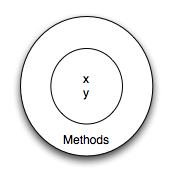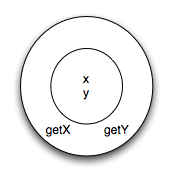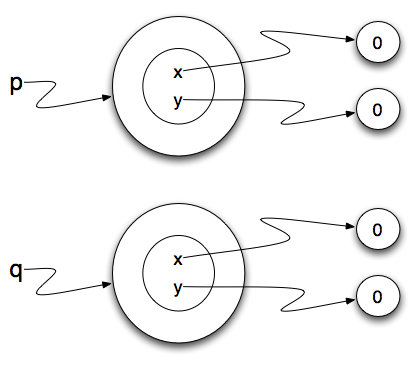# 17.4. User Defined Classes¶

We’ve already seen classes like `str`, `int`, `float` and `Turtle`. These were defined by Python and made available for us to use. However, in many cases when we are solving problems we need to create data objects that are related to the problem we are trying to solve. We need to create our own classes.

As an example, consider the concept of a mathematical point. In two dimensions, a point is two numbers (coordinates) that are treated collectively as a single object. Points are often written in parentheses with a comma separating the coordinates. For example, `(0, 0)` represents the origin, and `(x, y)` represents the point `x` units to the right and `y` units up from the origin. This `(x,y)` is the state of the point.

Thinking about our diagram above, we could draw a `point` object as shown here.Some of the typical operations that one associates with points might be to ask the point for its x coordinate, `getX`, or to ask for its y coordinate, `getY`. You may also wish to calculate the distance of a point from the origin, or the distance of a point from another point, or find the midpoint between two points, or answer the question as to whether a point falls within a given rectangle or circle. We’ll shortly see how we can organize these together with the data.Now that we understand what a `point` object might look like, we can define a new class. We’ll want our points to each have an `x` and a `y` attribute, so our first class definition looks like this.

 ```1 2 3 4 5 6 7``` ```class Point: """ Point class for representing and manipulating x,y coordinates. """ def __init__(self): """ Create a new point at the origin """ self.x = 0 self.y = 0 ```

Class definitions can appear anywhere in a program, but they are usually near the beginning (after the `import` statements). The syntax rules for a class definition are the same as for other compound statements. There is a header which begins with the keyword, `class`, followed by the name of the class, and ending with a colon.

If the first line after the class header is a string, it becomes the docstring of the class, and will be recognized by various tools. (This is also the way docstrings work in functions.)

Every class should have a method with the special name `__init__`. This initializer method, often referred to as the constructor, is automatically called whenever a new instance of `Point` is created. It gives the programmer the opportunity to set up the attributes required within the new instance by giving them their initial state values. The `self` parameter (you could choose any other name, but nobody ever does!) is automatically set to reference the newly-created object that needs to be initialized.

So let’s use our new Point class now.

During the initialization of the objects, we created two attributes called x and y for each, and gave them both the value 0.

Note

The asignments are not to `x` and `y`, but to `self.x` and `self.y`. The attributes `x` and `y` are always attached to a particular instance. The instance is always explicitly referenced with dot notation.

You will note that when you run the program, nothing happens. It turns out that this is not quite the case. In fact, two `Points` have been created, each having an x and y coordinate with value 0. However, because we have not asked the point to do anything, we don’t see any other result.You can see this for yourself, via codelens:

Activity: CodeLens 17.4.2 (chp13_points)

The following program adds a few print statements. You can see that the output suggests that each one is a `Point object`. However, notice that the `is` operator returns `False` meaning that they are different objects (we will have more to say about this in a later chapter).

This should look familiar — we’ve used classes before to create more than one object:

```from turtle import Turtle

tess = Turtle()     # Instantiate objects of type Turtle
alex = Turtle()
```

The variables `p` and `q` are assigned references to two new `Point` objects. A function like `Turtle` or `Point` that creates a new object instance is called a constructor. Every class automatically uses the name of the class as the name of the constructor function. The definition of the constructor function is done when you write the `__init__` function.

It may be helpful to think of a class as a factory for making objects. The class itself isn’t an instance of a point, but it contains the machinery to make point instances. Every time you call the constructor, you’re asking the factory to make you a new object. As the object comes off the production line, its initialization method is executed to get the object properly set up with its factory default settings.

The combined process of “make me a new object” and “get its settings initialized to the factory default settings” is called instantiation.